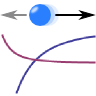## Drude model: Deriving the Conductivity and Permittivity of MetalsThe Drude model explains the electrodynamic properties of metals. The simple approach is to regard the conduction band electrons as non-interacting electron gas and yields a fairly accurate description of metals like silver, gold or aluminium.

## Problem StatementWithin the Drude model, we assume that electrons with mass $$m_{e}$$, charge $$-e$$ and density $$n_e$$ are influenced by an external electric field $$\mathbf{E}\left(t\right)$$ but obey negligible mutual interaction (electron gas).

Find the equations of motion and incorporate all inner-metallic processes like collisions to the nuclei into a friction term.

Solve the equations of motion in Fourier (frequency) space and determine the susceptibility $$\chi\left(\omega\right)$$ and permittivity $$\varepsilon\left(\omega\right)$$ of the Drude model electron gas.

Now use Ohm's law to further derive the conductivity $$\sigma\left(\omega\right)$$ and verify that $$\chi\left(\omega\right)=\mathrm{i}\sigma\left(\omega\right)/\omega$$.

##Background: The Drude Model as a Working Horse in Theoretical Electrodynamics

Now we have derived the Drude model dispersion relation which was quite easy once we went into Fourier space. Now the main questions are, if such a dispersion relation can really reflect the electrodynamic properties of real metals and how this description may be useful.

On the right you can see a comparison of the dispersion relation of silver taken from Johnson and Christy's "Optical Constants of the Noble Metals", Phys. Rev. B 6 (1972), pdf. What we can see is that we find a very good agreement of the data to a Drude model fit, both for real and imaginary part of the relative permittivity $$\varepsilon_r$$. Optical frequencies are decoded by their very colors.

The agreement to experimental data leads to a boiling down of the whole dispersion relation to just two parameters - plasma frequency $$\omega_\mathrm{p}$$ and loss rate $$\gamma$$. Such a simple theoretical description is a huge advantage compared to numerical data: Researchers can rely on the dispersion relation and derive other theories on top of the Drude description. This lead to a better understanding of certain metamaterial effects^  or of metal particles coupled to quantum systems^.. The Drude model is so widely applied that it is hard to find publications investigating light-metal-interactions that to not use it.

If you liked the simplicity of the Drude model, you can try to generalize it to the case when a magnetic field is present, see "The Dispersion Relation of a Magnetized Plasma". In this problem you will find that the magnetic field makes the response of the plasma strongly depends on the polarization of the electric field which will finally bring us to an understanding of the so-called Faraday rotation.